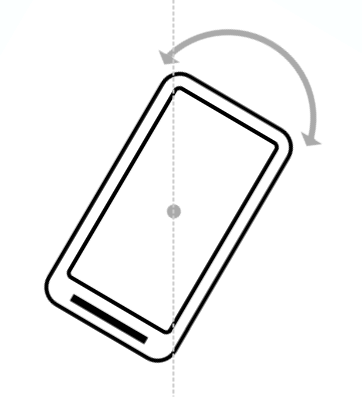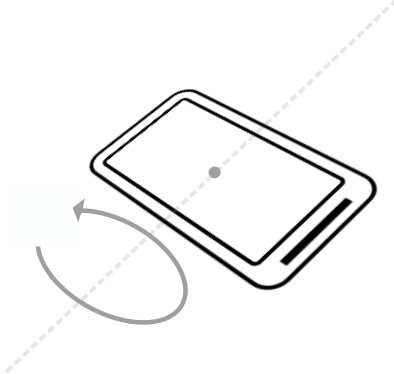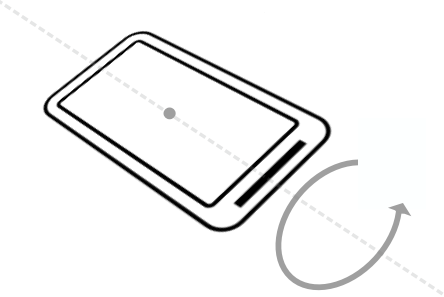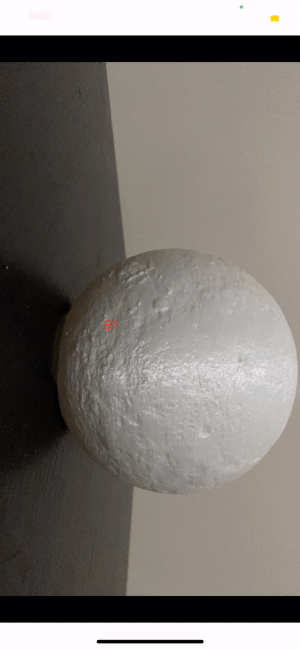## WorldMotion

Use a CoreMotion sensor to use a coordinate system that represents device motion or position relative to the Earth. In this coordinate system:

• y points to magnetic north along the surface of the Earth.
• x is 90 degrees from y , pointing approximately east.
• z extends up into space. Negative z extends down into the ground

## Determining device orientation

Device orientation is the position of the device in space relative to the Earth's coordinate system (specifically, to the magnetic north pole).

The `getRotationMatrix()` method generates a rotation matrix from the accelerometer and geomagnetic field sensor. A rotation matrix is a linear algebra concept that translates the sensor data from one coordinate system to another—in this case, from the device's coordinate system to the Earth's coordinate system.

The `getOrientation()` method uses the rotation matrix to compute the angles of the device's orientation. All of these angles are in radians, with values from -π to π. There are three components to orientation:

Azimuth : The angle between the device's current compass direction and magnetic north. If the top edge of the device faces magnetic north, the azimuth is 0.Pitch : The angle between a plane parallel to the device's screen and a plane parallel to the ground.Roll : The angle between a plane perpendicular to the device's screen and a plane perpendicular to the ground.Note: orientation angles use a different coordinate system than the one used in aviation (for yaw, pitch, and roll). In the aviation system, the x -axis is along the long side of the plane, from tail to nose.

# Use case

Let's assume that you want to know the angle of rotation around a certain object in the real world, so you need the value `x` from the Earth's coordinate system.

1. Convert a rotation vector to a rotation matrix.
``````  let matrixFromVector = rotationMatrix.getRotationMatrixFromVector()
``````
1. Rotating the supplied rotation matrix so it is expressed in a different coordinate system using.
`````` let coordinateMatrix = matrixFromVector.coordinateSystem(CMRotationMatrix.axisX, CMRotationMatrix.axisY)
``````
1. Compute the device's orientation based on the rotation matrix.
`````` let acceleration = coordinateMatrix.getOrientation()
``````

The return values is a radius that needs to be converted into degrees using `toDegrees`

##### Output## Using

• EarthCoordinate:
``````let earthCoordinate = EarthCoordinate()
// .......
deinit {
earthCoordinate.stop()
}
override func viewDidAppear(_ animated: Bool) {
super.viewDidAppear(animated)

earthCoordinate.delegate = self
earthCoordinate.start(interval: 0.2, queue: .init())

}

// MARK: -  EarthCoordinate delegate

extension ViewController: EarthCoordinateDelegate {

func onOrientationChange(x: Double, y: Double, z: Double) {
// ..... calculate value degrees

}
}

``````
• RotationMatrix
``````
let rotationMatrix = deviceMotion.attitude.rotationMatrix
let matrixFromVector = rotationMatrix.getRotationMatrixFromVector()
let coordinateMatrix = safeSelf.getCoordinateSystem(with: matrixFromVector)
let acceleration = coordinateMatrix.getOrientation()
``````

## Example

To run the example project, clone the repo, and run `pod install` from the Example directory first.

• Swift 4.2+
• Xcode 10.0+
• iOS 11.0+

## Installation

WorldMotion is available through CocoaPods. To install
it, simply add the following line to your Podfile:

``````pod 'WorldMotion'
``````

## Author

Ahmad Almasri, [email protected]

## GitHub

https://github.com/ahmedAlmasri/WorldMotion